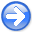Time-shift imaging condition in seismic migrationNext: Time-shift imaging in Kirchhoff Up: Time-shift imaging condition in Previous: Time-shift imaging condition

# Moveout analysishyper
Figure 3.
An image is formed when the Kirchoff stacking curve (dashed line) touches the true reflection response. Left: the case of under-migration; right: over-migration.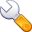off
Figure 4.
Common-image gathers for space-shift imaging (left column) and time-shift imaging (right column).ssk
Figure 5.
Common-image gathers after slant-stack for space-shift imaging (left column) and for time-shift imaging (right column). The vertical line indicates the migration velocity.We can use the Kirchhoff formulation to analyze the moveout behavior of the time-shift imaging condition in the simplest case of a flat reflector in a constant-velocity medium (Figures 3-5).

The synthetic data are imaged using shot-record wavefield extrapolation migration. Figure 4 shows offset common-image gathers for three different migration slownesses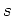, one of which is equal to the modeling slowness. The left column corresponds to the space-shift imaging condition and the right column corresponds to the time-shift imaging condition.

For the space-shift CIGs imaged with correct slowness, left column in Figure 4, the energy is focused at zero offset, but it spreads in a region of offsets when the slowness is wrong. Slant-stacking produces the images in left column of Figure 5.

For the time-shift CIGs imaged with correct slowness, right column in Figure 4, the energy is distributed along a line with a slope equal to the local velocity at the reflector position, but it spreads around this region when the slowness is wrong. Slant-stacking produces the images in the right column of Figure 5.

Letandrepresent the true slowness and reflector depth, andandstand for the corresponding quantities used in migration. An image is formed when the Kirchoff stacking curvetouches the true reflection response(Figure 3). Solving forfrom the envelope conditionyields two solutions:(27)

and(28)

Substituting solutions 27 and 28 in the conditionproduces two images in thespace. The first image is a straight line(29)

and the second image is a segment of the second-order curve(30)

Applying a slant-stack transformation withturns line (29) into a pointin thespace, while curve (30) turns into the curve(31)

The curvature of thecurve atis a clear indicator of the migration velocity errors.

By contrast, the moveout shapeappearing in wave-equation migration with the lateral-shift imaging condition is (Bartana et al., 2005)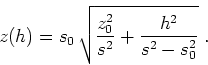(32)

After the slant transformation, the moveout curve (32) turns into the curve(33)

which is applicable for velocity analysis. A formal connection between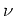-parameterization in equation (31) and-parameterization in equation (33) is given by(34)

or(35)

where. Equation (35) is a special case of equation (23) for flat reflectors. Curves of shape (31) and (33) are plotted on top of the experimental moveouts in Figure 5.Time-shift imaging condition in seismic migrationNext: Time-shift imaging in Kirchhoff Up: Time-shift imaging condition in Previous: Time-shift imaging condition

2007-04-08